U.S. Geological Survey Data Series 611

# 10CCT01 Subbottom Profiles

#### Home | Acronyms | Contents | Navigation | Profiles | Logs | Metadata | Software

10CCT01 | 10CCT02 | 10CCT03

Below are thumbnail images of each 10CCT01 trackline with links to full-size printable and geospatially interactive subbottom profiles. The printable profiles are GIF images that were gained using Seismic Unix software. These images are stored in the printable folder. Refer to the Software page for details about the processing and examples of the processing scripts. The processed SEG-Y data were exported to CTI SonarWeb software to produce the geospatially interactive profiles that allow the user to obtain a geographic location and depth for a given cursor position on the profile. This information is displayed in the status bar of the browser. The geospatial profiles, which are stored in the geospatial folder, have been corrected for the offset between the shotpoint and GPS antenna (fig. 1).

Figure 3 shows the trackline locations of the geospatial and printable profiles presented below.

Table 2 lists trackline statistics for 10CCT01.

NOTE: As a result of an unknown software malfunction during cruise 10CCT01, no data were recorded for line 10c19a. Lines 10c26 and 10c27 were corrupted for a short period of time by an anomaly in the Chirp data acquisition hardware, the Edgetech 3200-XS (Model: SB-424) subbottom profiling system. This disruption took the form of data/time/navigation jumps in both lines and was most likely caused by momentary system power glitches of the SB-424 and associated network hard drive.

NOTE: Please see the Home page for information about browser setting requirements to properly view the geospatial profiles. Depths shown on the geospatial profiles and two-way travel (TWT) times shown on the printable profile images are relative to the towfish, not to the sea surface

Line: 10c01  6776 traces
Start of line:   10c01     SHOT=1  day=73 hour=16  minute=44  sec=22
End of line:   10c01  SHOT=6776  day=73 hour=17  minute=12  sec=36

GEOSPATIAL | PRINTABLE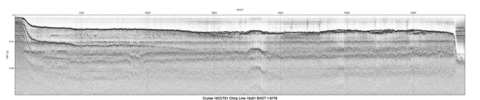Line: 10c02  7299 traces
Start of line:   10c02     SHOT=1  day=73 hour=17  minute=14  sec=24
End of line:   10c02  SHOT=7299  day=73 hour=17  minute=44  sec=48

GEOSPATIAL | PRINTABLE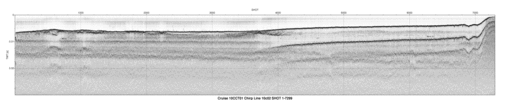Line: 10c03  6080 traces
Start of line:   10c03     SHOT=1  day=73 hour=17  minute=51  sec=30
End of line:   10c03  SHOT=6080  day=73 hour=18  minute=16  sec=50

GEOSPATIAL | PRINTABLE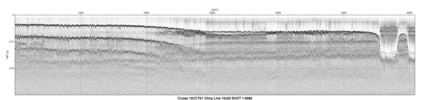Line: 10c04  8855 traces
Start of line:   10c04     SHOT=1  day=73 hour=18  minute=18  sec=57
End of line:   10c04  SHOT=8855  day=73 hour=18  minute=55  sec=50

GEOSPATIAL | PRINTABLE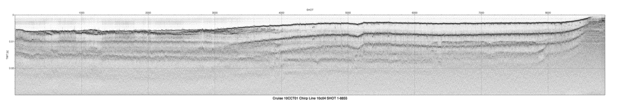Line: 10c05  2296 traces
Start of line:   10c05     SHOT=1  day=73 hour=18  minute=58  sec=38
End of line:   10c05  SHOT=2296  day=73 hour=19   minute=8  sec=12

GEOSPATIAL | PRINTABLE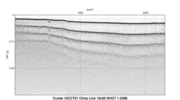Line: 10c06  7379 traces
Start of line:   10c06     SHOT=1  day=73 hour=19  minute=10   sec=2
End of line:   10c06  SHOT=7379  day=73 hour=19  minute=40  sec=47

GEOSPATIAL | PRINTABLE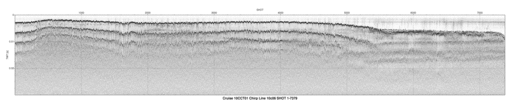Line: 10c07  2065 traces
Start of line:   10c07     SHOT=1  day=73 hour=19  minute=44  sec=26
End of line:   10c07  SHOT=2065  day=73 hour=19  minute=53   sec=2

GEOSPATIAL | PRINTABLE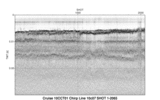Line: 01c08  8260 traces
Start of line:   01c08     SHOT=1  day=74 hour=15  minute=16  sec=15
End of line:   01c08  SHOT=8260  day=74 hour=15  minute=50  sec=40

GEOSPATIAL | PRINTABLE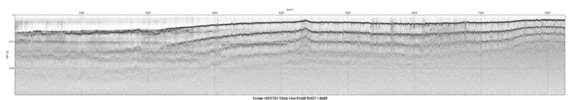Line: 01c09  1830 traces
Start of line:   01c09     SHOT=1  day=74 hour=15  minute=51  sec=49
End of line:   01c09  SHOT=1830  day=74 hour=15  minute=59  sec=26

GEOSPATIAL | PRINTABLE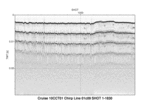Line: 01c10  7717 traces
Start of line:   01c10     SHOT=1  day=74 hour=15  minute=59  sec=39
End of line:   01c10  SHOT=7717  day=74 hour=16  minute=31  sec=48

GEOSPATIAL | PRINTABLE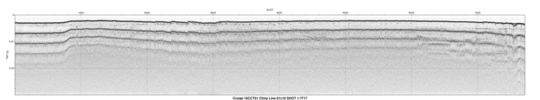Line: 01c11 13412 traces
Start of line:   01c11     SHOT=1  day=74 hour=16  minute=53   sec=0
End of line:   01c11 SHOT=13412  day=74 hour=17  minute=48  sec=53

GEOSPATIAL | PRINTABLE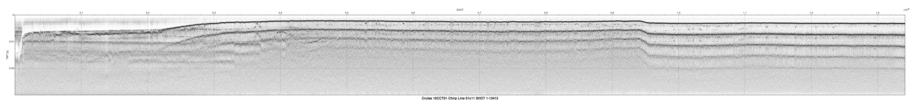Line: 10c12 12170 traces
Start of line:   10c12     SHOT=1  day=74 hour=17  minute=48  sec=55
End of line:   10c12 SHOT=12170  day=74 hour=18  minute=39  sec=37

GEOSPATIAL | PRINTABLE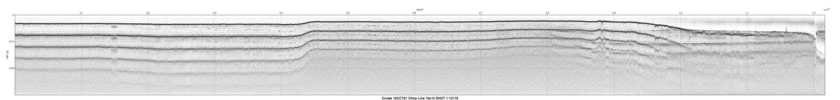Line: 10c13 12800 traces
Start of line:   10c13     SHOT=1  day=74 hour=18  minute=42  sec=19
End of line:   10c13 SHOT=12800  day=74 hour=19  minute=35  sec=39

GEOSPATIAL | PRINTABLE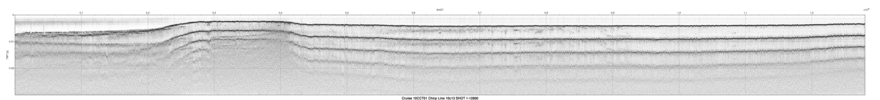Line: 10c14 12266 traces
Start of line:   10c14     SHOT=1  day=74 hour=19  minute=38   sec=2
End of line:   10c14 SHOT=12266  day=74 hour=20  minute=29   sec=9

GEOSPATIAL | PRINTABLE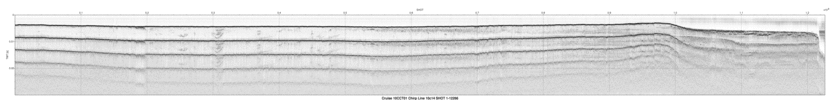Line: 10c15 13175 traces
Start of line:   10c15     SHOT=1  day=74 hour=20  minute=31  sec=41
End of line:   10c15 SHOT=13175  day=74 hour=21  minute=26  sec=34

GEOSPATIAL | PRINTABLE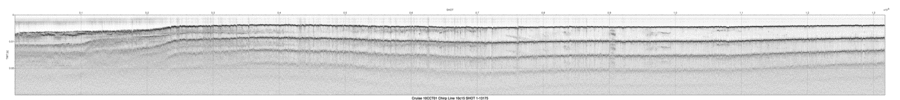Line: 10c16 15877 traces
Start of line:   10c16     SHOT=1  day=74 hour=21  minute=32  sec=23
End of line:   10c16 SHOT=15877  day=74 hour=22  minute=38  sec=32

GEOSPATIAL | PRINTABLE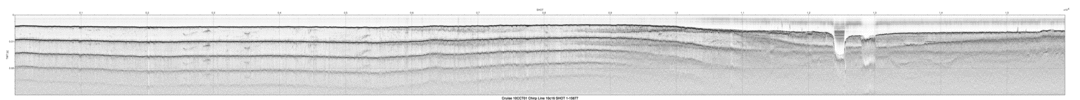Line: 10c17 16373 traces
Start of line:   10c17     SHOT=1  day=75 hour=14   minute=7   sec=6
End of line:   10c17 SHOT=16373  day=75 hour=15  minute=15  sec=19

GEOSPATIAL | PRINTABLE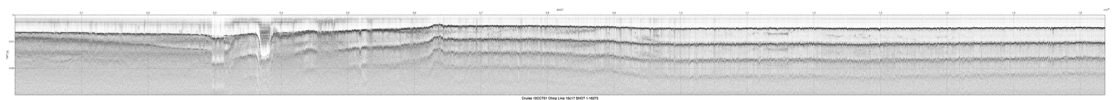Line: 10c18 16525 traces
Start of line:   10c18     SHOT=1  day=75 hour=15  minute=21  sec=54
End of line:   10c18 SHOT=16525  day=75 hour=16  minute=30  sec=45

GEOSPATIAL | PRINTABLE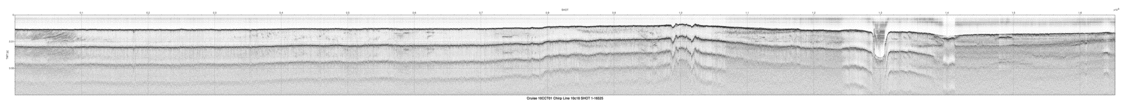Line: 10c19  6256 traces
Start of line:   10c19     SHOT=1  day=75 hour=16  minute=35  sec=42
End of line:   10c19  SHOT=6256  day=75 hour=17   minute=1  sec=46

GEOSPATIAL | PRINTABLE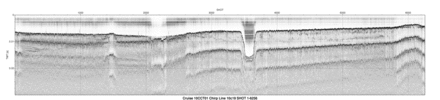Line: 10c19b  3940 traces
Start of line:  10c19b     SHOT=1  day=75 hour=17  minute=15  sec=49
End of line:  10c19b  SHOT=3940  day=75 hour=17  minute=32  sec=14

GEOSPATIAL | PRINTABLE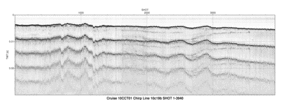Line: 10c20 11475 traces
Start of line:   10c20     SHOT=1  day=75 hour=17  minute=39  sec=18
End of line:   10c20 SHOT=11475  day=75 hour=18  minute=27   sec=6

GEOSPATIAL | PRINTABLE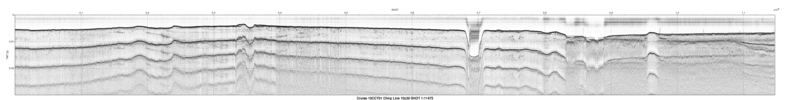Line: 10c21 11339 traces
Start of line:   10c21     SHOT=1  day=75 hour=18  minute=30   sec=6
End of line:   10c21 SHOT=11339  day=75 hour=19  minute=17  sec=20

GEOSPATIAL | PRINTABLE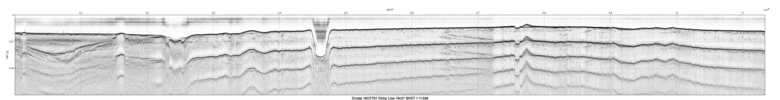Line: 10c22 10473 traces
Start of line:   10c22     SHOT=1  day=75 hour=19  minute=20  sec=15
End of line:   10c22 SHOT=10473  day=75 hour=20   minute=3  sec=53

GEOSPATIAL | PRINTABLE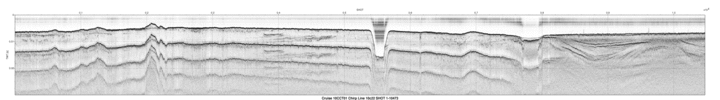Line: 10c23 12694 traces
Start of line:   10c23     SHOT=1  day=75 hour=20   minute=8  sec=15
End of line:   10c23 SHOT=12694  day=75 hour=21   minute=1   sec=8

GEOSPATIAL | PRINTABLE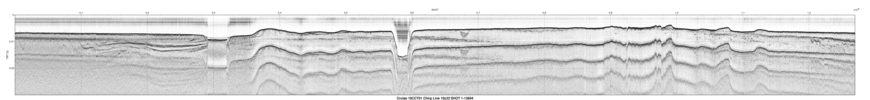Line: 10c24 11631 traces
Start of line:   10c24     SHOT=1  day=75 hour=21   minute=2  sec=56
End of line:   10c24 SHOT=11631  day=75 hour=21  minute=51  sec=23

GEOSPATIAL | PRINTABLE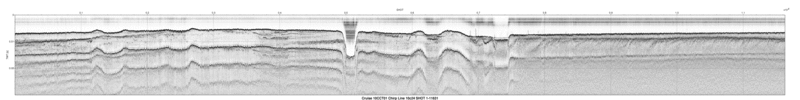Line: 10c25  6685 traces
Start of line:   10c25     SHOT=1  day=75 hour=21  minute=56  sec=42
End of line:   10c25  SHOT=6685  day=75 hour=22  minute=24  sec=33

GEOSPATIAL | PRINTABLE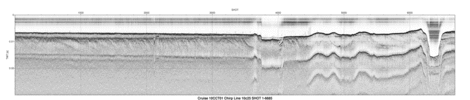Line: 10c26 12670 traces
Start of line:   10c26     SHOT=1  day=76 hour=21  minute=25   sec=1
End of line:   10c26 SHOT=12670  day=76 hour=14  minute=45  sec=22

GEOSPATIAL | PRINTABLE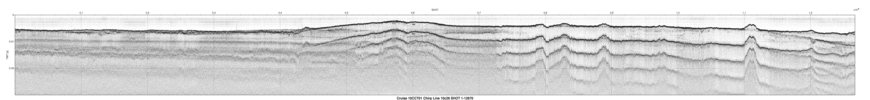Line: 10c26a  2442 traces
Start of line:  10c26a     SHOT=1  day=76 hour=21  minute=25   sec=1
End of line:  10c26a  SHOT=2442  day=76 hour=14   minute=2  sec=45

GEOSPATIAL | PRINTABLE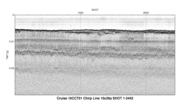Line: 10c27  3876 traces
Start of line:   10c27     SHOT=1  day=76 hour=14  minute=49  sec=52
End of line:   10c27  SHOT=3876  day=76 hour=13  minute=54  sec=35

GEOSPATIAL | PRINTABLE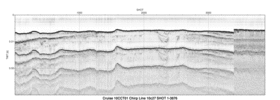Line: 10c27a  1241 traces
Start of line:  10c27a     SHOT=1  day=76 hour=15  minute=13  sec=18
End of line:  10c27a  SHOT=1241  day=76 hour=15  minute=18  sec=28

GEOSPATIAL | PRINTABLE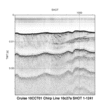Line: 10c28 10405 traces
Start of line:   10c28     SHOT=1  day=76 hour=15  minute=18  sec=50
End of line:   10c28 SHOT=10405  day=76 hour=16   minute=2  sec=11

GEOSPATIAL | PRINTABLE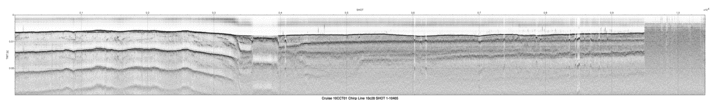Line: 10c29  2681 traces
Start of line:   10c29     SHOT=1  day=76 hour=16   minute=2  sec=25
End of line:   10c29  SHOT=2681  day=76 hour=16  minute=13  sec=35

GEOSPATIAL | PRINTABLE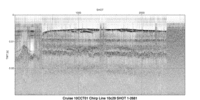Line: 10c30  9281 traces
Start of line:   10c30     SHOT=1  day=76 hour=16  minute=35  sec=14
End of line:   10c30  SHOT=9281  day=76 hour=17  minute=13  sec=54

GEOSPATIAL | PRINTABLE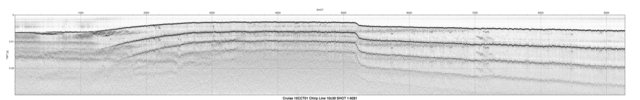Line: 10c31  4571 traces
Start of line:   10c31     SHOT=1  day=76 hour=17  minute=14   sec=3
End of line:   10c31  SHOT=4571  day=76 hour=17  minute=33   sec=5

GEOSPATIAL | PRINTABLE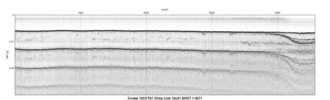Line: 10c32 10683 traces
Start of line:   10c32     SHOT=1  day=76 hour=17  minute=33  sec=16
End of line:   10c32 SHOT=10683  day=76 hour=18  minute=17  sec=46

GEOSPATIAL | PRINTABLE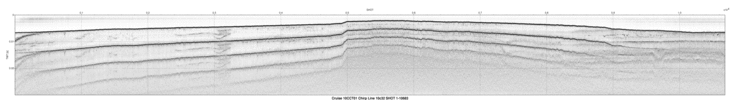Line: 10c33  1191 traces
Start of line:   10c33     SHOT=1  day=76 hour=18  minute=18  sec=21
End of line:   10c33  SHOT=1191  day=76 hour=18  minute=23  sec=19

GEOSPATIAL | PRINTABLE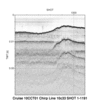Line: 10c34  7582 traces
Start of line:   10c34     SHOT=1  day=76 hour=18  minute=23  sec=26
End of line:   10c34  SHOT=7582  day=76 hour=18  minute=55   sec=2

GEOSPATIAL | PRINTABLE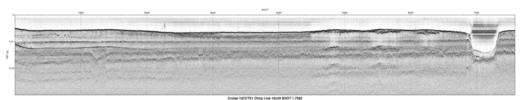Line: 10c35 12544 traces
Start of line:   10c35     SHOT=1  day=76 hour=18  minute=56  sec=53
End of line:   10c35 SHOT=12544  day=76 hour=19  minute=49   sec=9

GEOSPATIAL | PRINTABLE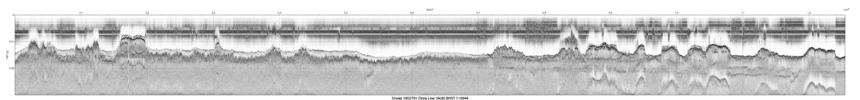Line: 10c36  4552 traces
Start of line:   10c36     SHOT=1  day=76 hour=20   minute=2  sec=18
End of line:   10c36  SHOT=4553  day=76 hour=20  minute=21  sec=16

GEOSPATIAL | PRINTABLE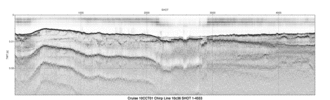#### Home | Acronyms | Contents | Navigation | Profiles | Logs | Metadata | SoftwareU.S. Department of the Interior | U.S. Geological Survey
URL: https://pubs.usgs.gov/ds/611/html/profiles.html